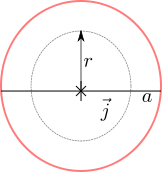(No Ratings Yet)Loading ...

# GATE-2012 ECE Q52 (electromagnetics)

by on December 1, 2012

Question 52 on electromagnetics from GATE (Graduate Aptitude Test in Engineering) 2012 Electronics and Communication Engineering paper.

## (D)  for  and  for## Solution

To answer this question on magnetic field, we need to determine the magnetic field inside and outside the cable using Ampere’s Law.

## Ampere’s Law :

The total current inside a closed curve  is the line integral of the magnetic field  (in Tesla)

,

where

is the magnetic field (in Tesla)

is the vector representing the infinitesimal line on the closed loop ,

is the net current enclosed by the closed loop and

is the permeability of vacuum.

Let us use this result to find the magnetic field in a uniform solid wire of radius  .

## Magnetic field in the region :Figure : Solid wire showing the imaginary Amperian loop inside the wire (circle with radius  )

Given that the current density is , the current through the area in the circle with radius is

Applying Ampere’s law,

,

where

is the permeability ( is the permeability of vacuum.  is the permeability of the material).

Given that the magnetic field is  is parallel to the line  and is uniform across the closed loop, it can be moved out of the integral, i.e

.

The term  is the circumference of the circle with radius  i.e.

.

Substituting, the magnetic field in the region  is,

.

## Magnetic field in the region :Figure : Solid wire showing the imaginary Amperian loop outside the wire (circle with radius  )

Given that the current density is , the current through the area in the circle with radius  is determined only by the current flowing through the cable with in the radius , i.e.

Applying Ampere’s law,

,

Taking  outside the intergral and substituting for the term  ,

The magnetic field in the region  is,

.

Summarizing,

Based on the above, the right choice is (C) i.e.  The magnetic field at a distance  from the center of the wire is proportional to  for  and  for

## References

 GATE Examination Question Papers [Previous Years] from Indian Institute of Technology, Madras http://gate.iitm.ac.in/gateqps/2012/ec.pdf

 Fields and Waves in Communication Electronics, Simon Ramo, John R. Whinnery, Theodore Van Duzer (buy from Amazon.combuy from Flipkart.com).

 The youtube videos uploaded by user lasseviren1 aided me in understanding the  integrals in electric field and magnetic field.

 Ampere’s Law

 Permeability

D id you like this article? Make sure that you do not miss a new article by subscribing to RSS feed OR subscribing to e-mail newsletter. Note: Subscribing via e-mail entitles you to download the free e-Book on BER of BPSK/QPSK/16QAM/16PSK in AWGN.

{ 0 comments… add one now }

Previous post:

Next post: